This tutorial will demonstrate how to lookup from another worksheet or spreadsheet using XLOOKUP in Excel. If your version of Excel does not support XLOOKUP, read how to use the VLOOKUP instead.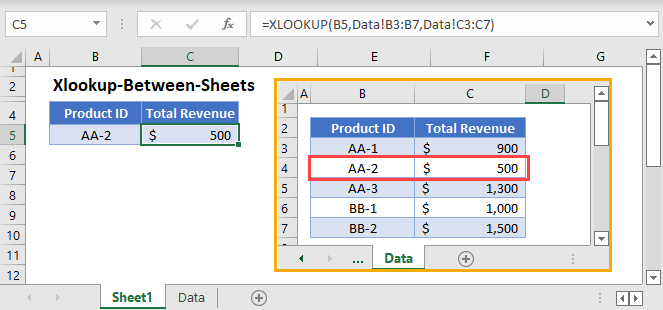## XLOOKUP from Another Worksheet

To reference a range from a different sheet, click the tab name at the bottom of Excel and select your range. Excel will automatically add the appropriate cell reference: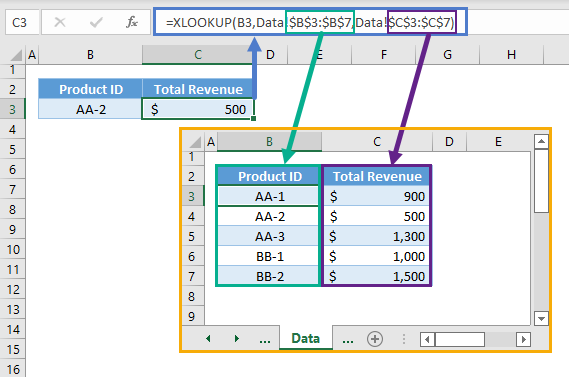If you’d like, you can manually type the cell reference. Notice how it’s displayed above.

However, this reference format will not work if there are spaces or special characters. If there are spaces or special characters, the cell reference will look like this instead (notice the apostrophes):

``=XLOOKUP(B3,'Data - 2'!\$B\$3:\$B\$7,'Data - 2'!\$C\$3:\$C\$7)``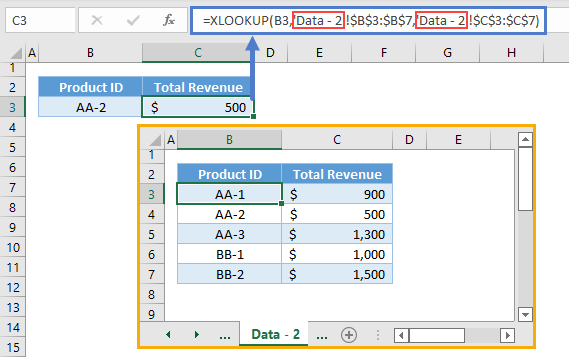## XLOOKUP from Another Workbook

If the data set is stored in a sheet from another workbook, we must also add the workbook reference.

``=XLOOKUP(B3,[Workbook2.xlsx]Data!\$B\$3:\$B\$7,[Workbook2.xlsx]Data!\$C\$3:\$C\$7)``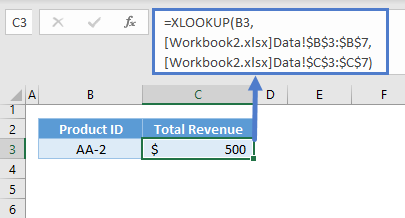If the workbook is open, only the workbook name will be displayed. When the workbook is closed, the full path will be shown:

``=XLOOKUP(B3,'D:\AUTOMATE EXCEL\[Workbook2.xlsx]Data'!\$B\$3:\$B\$7,'D:\AUTOMATE EXCEL\[Workbook2.xlsx]Data'!\$C\$3:\$C\$7)``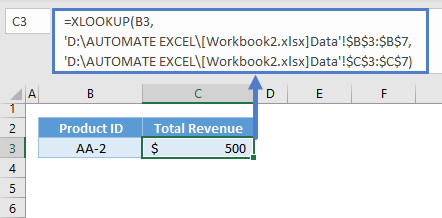### Excel Practice Worksheet

Practice Excel functions and formulas with our 100% free practice worksheets!